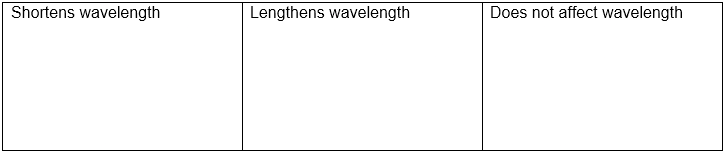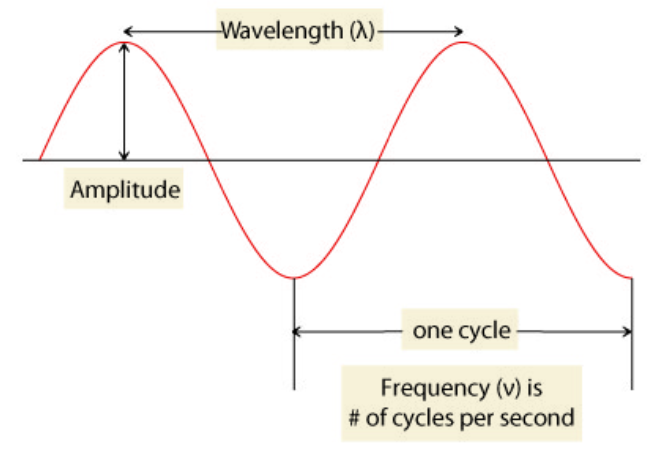# Problem: Part A. Classify each change (which can be manipulated within the green box) according to its effect on the wavelength.increase frequency          increase amplitude          increase dampingdecrease frequency         decrease amplitude        decrease dampingDrag the appropriate items to their respective bins.Light is a type of electromagnetic radiation, and light exhibits wave-particle behavior. The particle nature of light encompasses the concept of photons being specific packets of energy. Here, we are going to examine the wave nature of light. A beam of light has an oscillating magnetic field that is continuously perpendicular to an oscillating electric field, and the wave created by these fields can be described by the same properties of any other type of wave. The following image summarizes the properties of wavelength, frequency, and amplitude:Wavelength is the distance between crests, the amplitude is the distance between the baseline and the crest, and frequency is the number of cycles that are completed per unit of time, typically seconds, as s − 1 or Hz. Shorter wavelengths and higher intensity both correspond to higher energy. However, amplitude and wavelength are completely independent of each other. The wavelength can directly describe the energy of an individual photon (particle nature of light), whereas the amplitude would describe the number of photons existing at that part of the wave. Therefore, the intensity or amplitude of light is dependent on how many photons are in phase while in motion. Since light moves at a constant speed (c), frequency (ν) and wavelength (λ) are dependent on each other, and their relationship can be described by the following equation:c = λν

###### FREE Expert Solution
87% (368 ratings)View Complete Written Solution
###### Problem Details

Part A. Classify each change (which can be manipulated within the green box) according to its effect on the wavelength.

increase frequency          increase amplitude          increase damping
decrease frequency         decrease amplitude        decrease dampingDrag the appropriate items to their respective bins.

Light is a type of electromagnetic radiation, and light exhibits wave-particle behavior. The particle nature of light encompasses the concept of photons being specific packets of energy. Here, we are going to examine the wave nature of light. A beam of light has an oscillating magnetic field that is continuously perpendicular to an oscillating electric field, and the wave created by these fields can be described by the same properties of any other type of wave. The following image summarizes the properties of wavelength, frequency, and amplitude:Wavelength is the distance between crests, the amplitude is the distance between the baseline and the crest, and frequency is the number of cycles that are completed per unit of time, typically seconds, as s − 1 or Hz. Shorter wavelengths and higher intensity both correspond to higher energy. However, amplitude and wavelength are completely independent of each other. The wavelength can directly describe the energy of an individual photon (particle nature of light), whereas the amplitude would describe the number of photons existing at that part of the wave. Therefore, the intensity or amplitude of light is dependent on how many photons are in phase while in motion. Since light moves at a constant speed (c), frequency (ν) and wavelength (λ) are dependent on each other, and their relationship can be described by the following equation:

c = λν